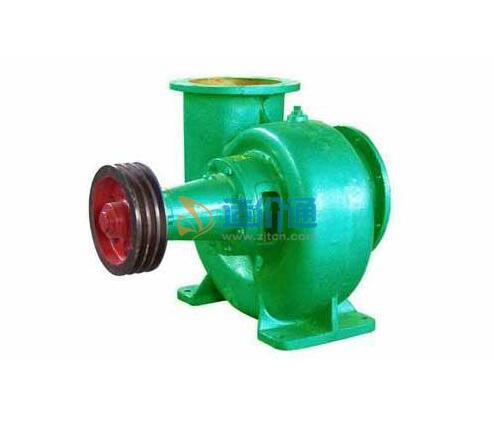[切换站点]

## 材料分类

400GHW-7 Q=1260m3/H,H=6.8m,N=30KW• 400GHW-7 Q=1260m3/H,H=6.8m,N=30KW
13% 安国市怡宝水泵经销处
2019-05

400GHW-7 Q=1260m3/h H=6.8m N=30KW• 400GHW-7 Q=1260m3/h H=6.8m N=30KW
13% 安国市怡宝水泵经销处
2019-05

500GHW-6 Q=1980m3/H,H=6.2m,N=55KW• 500GHW-6 Q=1980m3/H,H=6.2m,N=55KW
13% 安国市怡宝水泵经销处
2019-05

500GHW-6 Q=1980m3/h H=6.2m N=55KW• 500GHW-6 Q=1980m3/h H=6.2m N=55KW
13% 安国市怡宝水泵经销处
2019-05